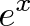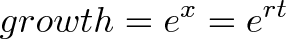## Posts Tagged 'e'

### eI spent some time last night reviewing some math fundamentals in an attempt to get my brain moved from el mundo de Español and back into the world of functions, algorithms, and the basics of calculus. You should know, too, that I have spent the summer collecting urls for math sites that might be of some use to me as I travel deeper in the abstraction jungle. One site last night was particularly helpful…..on the subject of e, the mathematical constant. If you are like me, you might ask, what is e? And, what is it good for? (Not absolutely nothing…..The song. Sorry….)

Believe me when I tell you that my calculus textbook is worthless in terms of an explanation. For a simpler (?) explanation, let’s go to Wikipedia:

The mathematical constant e is the unique real number such that the function ex has the same value as the slope of the tangent line, for all values of x. More generally, the only functions equal to their own derivatives are of the form Cex, where C is a constant. The function ex so defined is called the exponential function, and its inverse is the natural logarithm, or logarithm to base e. The number e is also commonly defined as the base of the natural logarithm (using an integral to define the latter), as the limit of a certain sequence, or as the sum of a certain seriesrepresentations of e, below). (see

The number e is one of the most important numbers in mathematics, alongside the additive and multiplicative identities 0 and 1, the constant π, and the imaginary unit i.

The number e is sometimes called Euler’s number after the Swiss mathematician Leonhard Euler. (e is not to be confused with γ – the Euler–Mascheroni constant, sometimes called simply Euler’s constant.)

Since e is transcendental, and therefore irrational, its value cannot be given exactly as a finite or eventually repeating decimal. The numerical value of e truncated to 20 decimal places is:

2.71828 18284 59045 23536…e is the unique number a, such that the value of the derivative (the slope of the tangent line) of the exponential function f (x) = ax (blue curve) at the point x = 0 is exactly 1. For comparison, functions 2x (dotted curve) and 4x (dashed curve) are shown; they are not tangent to the line of slope 1 (red).

Got that?

Good, neither did I.

But this guy does, and does a helluva job explaining it.

In a nutshell:

e is the base amount of growth shared by all continually growing processes. e lets you take a simple growth rate (where all change happens at the end of the year) and find the impact of compound, continuous growth, where every nanosecond (or faster) you are growing just a little bit.

e shows up whenever systems grow exponentially and continuously: population, radioactive decay, interest calculations, and more. Even jagged systems that don’t grow smoothly can be approximated by e.

Just like every number can be considered a “scaled” version of 1 (the base unit), every circle can be considered a “scaled” version of the unit circle (radius 1), and every rate of growth can be considered a “scaled” version of e (the “unit” rate of growth).

So e is not an obscure, seemingly random number. e represents the idea that all continually growing systems are scaled versions of a common rate.

Now we’re getting somewhere. I understand the principle of compound interest. Who knew it came from calculus?

To continue:

Why not take even shorter time periods? How about every month, day, hour, or even nanosecond? Will our returns skyrocket?

Our return gets better, but only to a point. Try using different numbers of n in our magic formula to see our total return:


n          (1 + 1/n)^n
------------------
1          2
2          2.25
3          2.37
5          2.488
10         2.5937
100        2.7048
1,000      2.7169
10,000     2.71814
100,000    2.718268
1,000,000  2.7182804
...



The numbers get bigger and converge around 2.718. Hey… wait a minute… that looks like e!

Yowza. In geeky math terms, e is defined to be that rate of growth if we continually compound 100% return on smaller and smaller time periods:This limit appears to converge, and there are proofs to that effect. But as you can see, as we take finer time periods the total return stays around 2.718.

## But what does it all mean?

The number e (2.718…) represents the compound rate of growth from a process that grows at 100% for one time period. Sure, you start out expecting to grow from 1 to 2. But with each tiny step forward you create a little “dividend” that starts growing on its own. When all is said and done, you end up with e (2.718…) at the end of 1 time period, not 2.

Now, of course, I understand why the bankers and financial advisers get so stoked about compound growth rates. This also gives me a peek behind the curtain as to why calculus offers so much to the rest of the natural sciences. Every discipline seeks answers about rates of change, and by golly, it sure does look like e helps them all measure those rates. To wrap things up…..

## The big secret: e merges rate and time.

This is wild! e^x can mean two things:

• x is the number of times we multiply a growth rate: 100% growth for 3 years is e^3
• x is the growth rate itself: 300% growth for one year is e^3.

Won’t this overlap confuse things? Will our formulas break and the world come to an end?

It all works out. When we write:the variable x is a combination of rate and time.Let me explain. When dealing with compound growth, 10 years of 3% growth has the same overall impact as 1 year of 30% growth (and no growth afterward).

• 10 years of 3% growth means 30 changes of 1%. These changes happen over 10 years, so you are growing continuously at 3% per year.
• 1 period of 30% growth means 30 changes of 1%, but happening in a single year. So you grow for 30% a year and stop.

The same “30 changes of 1%” happen in each case. The faster your rate (30%) the less time you need to grow for the same effect (1 year). The slower your rate (3%) the longer you need to grow (10 years).

But in both cases, the growth is e^.30 = 1.35 in the end. We’re impatient and prefer large, fast growth to slow, long growth but e shows they have the same net effect.

So, our general formula becomes:If we have a return of r for t time periods, our net compound growth is e^rt. This even works for negative and fractional returns, by the way.

## Example Time!

Examples make everything more fun. A quick note: We’re so used to formulas like 2^x and regular, compound interest that it’s easy to get confused (myself included). Read more about simple, compound and continuous growth.

These examples focus on smooth, continuous growth, not the “jumpy” growth that happens at yearly intervals. There are ways to convert between them, but we’ll save that for another article.

Example 1: Growing crystals

Suppose I have 300kg of magic crystals. They’re magic because they grow throughout the day: I watch a single crystal, and in the course of 24 hours it creates its own weight in crystals. (Those baby crystals start growing immediately as well, but I can’t track that). How much will I have after 10 days?

Well, since the crystals start growing immediately, we want continuous growth. Our rate is 100% every 24 hours, so after 10 days we get: 300 * e^(1 * 10) = 6.6 million kg of our magic gem.

Example 2: Maximum interest rates

Suppose I have $120 in a count with 5% interest. My bank is generous and gives me the maximum possible compounding. How much will I have after 10 years? Our rate is 5%, and we’re lucky enough to compound continuously. After 10 years, we get$120 * e^(.05 * 10) = \$197.85. Of course, most banks aren’t nice enough to give you the best possible rate. The difference between your actual return and the continuous one is how much they don’t like you.

Example 3: Radioactive decay

I have 10kg of a radioactive material, which appears to continuously decay at a rate of 100% per year. How much will I have after 3 years?

Zip? Zero? Nothing? Think again.

Decaying continuously at 100% per year is the trajectory we start off with. Yes, we do begin with 10kg and expect to “lost it all” by the end of the year, since we’re decaying at 10 kg/year.

We go a few months and get to 5kg. Half a year left? Nope! Now we’re losing at a rate of 5kg/year, so we have another full year from this moment!

We wait a few more months, and get to 2kg. And of course, now we’re decaying at a rate of 2kg/year, so we have a full year (from this moment). We get 1 kg, have a full year, get to .5 kg, have a full year — see the pattern?

As time goes on, we lose material, but our rate of decay slows down. This constantly changing growth is the essence of continuous growth & decay.

After 3 years, we’ll have 10 * e^(-1 * 3) = .498 kg. We use a negative exponent for decay — we want a fraction (1/ert) vs a growth multiplier (e(rt)). [Decay is commonly given in terms of “half life”, or non-continuous growth. We’ll talk about converting these rates in a future article.]

More Examples

If you want fancier examples, try the Black-Scholes option formula (notice e used for exponential decay in value) or radioactive decay. The goal is to see e^rt in a formula and understand why it’s there: it’s modeling a type of growth or decay.

And now you know why it’s “e”, and not pi or some other number: e raised to “r*t” gives you the growth impact of rate r and time t.

I think I understand e a little better now. How about you?